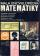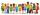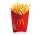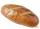Cows

20 cows has food for 60 days. We reduce the dose to two-thirds for 18 cows. How many days will be fed?

Result

x =  85.714

Solution:

20*60 = 18* (2/3)*x + 2x

42x = 3600

x = 6007 ≈ 85.714286

Calculated by our simple equation calculator.

Leave us a comment of this math problem and its solution (i.e. if it is still somewhat unclear...):Be the first to comment!To solve this verbal math problem are needed these knowledge from mathematics:

Do you have a linear equation or system of equations and looking for its solution? Or do you have quadratic equation?

Next similar math problems:

1. InfirmaryTwo thirds of children from the infirmary went on a trip seventh went to bathe and 40 children remained in the gym. How many children were treated in the infirmary?
2. How oldThe student who asked how many years he answered: "After 10 years I will be twice as old than as I was four years ago. How old is student?
3. BookTo number pages of thick book was used 4201 digits. How many pages has this book?
4. NormThree workers planted 3555 seedlings of tomatoes in one dey. First worked at the standard norm, the second planted 120 seedlings more and the third 135 seedlings more than the first worker. How many seedlings were standard norm?
5. The classroomIn the seventh class the number of boys and girls are at a ratio of 4:5. Boys are 16. How many total students are in the classroom?
6. QuizTested student answered correctly on a 4/ 8 questions. Wrong answers was 16. How many questions answered?
7. Tickets 3A total of 645 tickets were sold for the school play. They were either adult tickets or student tickets. There were 55 fewer student tickets sold than adult tickets. How many adult tickets were sold?
8. Forest nurseryIn the forest nursery after winter, they found that 1/10 stems died out of them. For them, they land 193 new spruces. How many spruces are in the forest nursery?
9. NovakNovak needed to dig up three of the same pit in the garden. The first pit dug father alone for 15 hours. His second dig son helped him and it did that in six hours. The third pit dug son himself. How long it took him?
10. Hectoliters of waterThe pool has a total of 126 hectoliters of water. The first pump draws 2.1 liters of water per second. A second pump pumps 3.5 liters of water per second. How long will it take both pumps to drain four-fifths of the water at the same time?
11. CaloriesA large order of McDonald’s french fries has 500 calories. Of this total, 220 calories are from fat. Find the ratio of the calories from fat to total calories in a large order of McDonald’s french fries.
12. Three paintersOne painter would have painted the fence for 15 hours, the second in 12 hours. The plot had to be painted in four hours, so they called the third one, and all worked together. For what time would the third painter paint fence the fence alone?5 of the same bread has the same weight as three bread and 4 kg of fruit. What weight has one bread?Seven times of my age is 8 less than the largest two-digit number. How old I am?Solve: if 2(x-1)=14, then x= (solve an equation with one unknown)2x + 3 + 7x = – 24, what is the value of x?Solve for x: 3(x + 2) = x - 18